Electron. J. Diff. Equ., Vol. 2010(2010), No. 21, pp. 1-18.

### Asymptotic stability of switching systems Driss Boularas, David Cheban

Abstract:
In this article, we study the uniform asymptotic stability of the switched system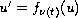,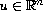, where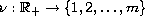is an arbitrary piecewise constant function. We find criteria for the asymptotic stability of nonlinear systems. In particular, for slow and homogeneous systems, we prove that the asymptotic stability of each individual equation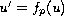(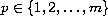) implies the uniform asymptotic stability of the system (with respect to switched signals). For linear switched systems (i.e.,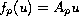, where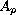is a linear mapping acting on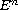) we establish the following result: The linear switched system is uniformly asymptotically stable if it does not admit nontrivial bounded full trajectories and at least one of the equations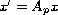is asymptotically stable. We study this problem in the framework of linear non-autonomous dynamical systems (cocyles).

Submitted December 21, 2009. Published February 2, 2010.
Math Subject Classifications: 34A37, 34D20, 34D23, 34D45, 37B55, 37C75, 93D20.
Key Words: Uniform asymptotic stability; cocycles; globalattractors; uniform exponential stability; switched systems.

Show me the PDF file (341 KB), TEX file, and other files for this article.Driss Boularas Xlim, UMR 6090, DMI, Faculté de Sciences Université de Limoges 123, Avenue A. Thomas 87060, Limoges, France email: driss.boularas@unilim.fr David Cheban State University of Moldova Department of Mathematics and Informatics A. Mateevich Street 60 MD-2009 Chisinau, Moldova email: cheban@usm.md